# The Straight Line and the circle

## (Note: All the images are interactive, move the points around to see. Dynamic mathematics interactive web page with Cinderella )

A moving point describes a straight line when it passes from one
position to another along the shortest possible path. A straight line
can be drawn with the help of a ruler; when a pencil runs along the
edge of a ruler it leaves a trace on the paper in the form of a
straight line.

When a point moves on a surface at a constant distance from another
fixed point on the same surface it describes a circle. Because of this
property of the circle we are able to draw a circle with the help of
compasses.

The straight line and the circle are the simplest and at the same time
the most remarkable curves as far as their properties are concerned.

You are no doubt more familiar with these two curves than with
others. But you should not imagine that you know all of the most
important properties of straight lines and curves. For example, you
may not know that if the vertices of the triangles $ABC$ and $AB’C’$
lie on three straight lines intersecting at the point $S$ (Fig. 1),
the three points of intersection $M$, $K$, $L$ of the corresponding
sides of the triangles, the sides $AB$ and $A’B’$, $BC$ and $B’C’$,
and $AC$ and $A’C’$, must be collinear, that is, they lie on a single
straight line.

(Note: this image below is interactive, move the points to see the dynamic change!)

You are sure to know that a point $M$ moving in a plane equidistantly
from two fixed points, say $F_1$, and $F_2$, of the same plane, that
is, so that $MF_{1}= MF_{2}$, describes a straight line (Fig. 2).

But you might find it difficult to answer the question:

What type of curve will point $M$ describe if the distance of $M$ from
$F_1$, is a certain number of times greater than that from $F_2$ (for
instance, in Fig. 3 it is twice as great)?

The curve turns out to be a circle. Hence if the point $M$ moves in a
plane so that the distance of $M$ from one of the fixed
points. $F_{1}$ or $F_{2}$, in the same plane is always proportional
to the distance from the other fixed point, that is

$$MF_{1} = k \times MF_{2}$$

then $M$ describes either a straight line (when the factor of
proportionality is unity) or a circle (when the factor of
proportionality is other than unity).

This is a post to create interactive mathematics elements using Cinderella a Free Software alternative to GeoGebra which is no longer a Free Software. The files have been exported from Cinderella at html interactives)

# Introduction

The Government of India are keenly interested in promoting the pattern of Basic education in the country and h ave adopted a number of measures for this purpose. These include programmes of expansion as well . as steps to improve the quality of work ·in Basic schools and their methods and techniques. If this
objective is to be achieved, it is essential that all those who work in the field should have a clear concept of what Basic education means and should be able to distinguish between its essential features and what are mainly matters of detail to b e adjusted in the light of local needs and developing experience.

This Statement about the Concept of Basic Education , which has been prepared by the Basic Education Standing Committee of the Central Advisory Board of Education, is meant to highlight its significant features and. to remove possible misunderstandings. I hope our educationists will ponder over it and try to work out the scheme in the spirit that it envisages, so that Basic education may play its proper role in inculcating the right attitudes and ideals of character and efficiency in our children.

# The Concept Of Basic Education

The term ‘Basic Education’ has been interpreted — and sometimes misinterpreted — in a. variety of ways. This is, to some extent understandable because it is a comparatively recent development and its concept and technique are still in the making. It seems necessary therefore, to state clearly what is meant by
Basic education.

Broadly speaking, it may be stated that the concept of Basic education is the same as defined in the Report of the Basic National Education Committee (the Zakir Husain Committee) and elucidated by the Central Advisory Board of Education. It is clear that the basic principles and techniques, as made out in that Report, should guide and shape educational reconstruction in India.So far as the provision of eight years of compulsory universal schooling and the use of the mother tongue as the medium of instruction are concerned, there is now no difference of opinion about them. They have come to be universally accepted and need no further elucidation, except in so far as it may be necessary to stress the intrinsic wholeness of the entire period of Basic education, covering the Junior as well as Senior Basic grades. The other implications and features of Basic education that need to be clarified and stressed are the following:

1. Basic education, as conceived and explained by Mahatma Gandhi, is essentially an education for life, and what· is more, an education through life. It aims at creating eventually a social order free from exploitation and violence. That is why productive, creative and socially useful work in which all boys and girls may. participate, irrespective of any distinction of caste or creed or class, is placed at the very centre of Basic education.
2. The effective teaching of a basic craft, thus, becomes an essential part of education at this stage, as productive work, done under proper conditions, not only makes the acquisition of much related knowledge more concrete and realistic but also adds a powerful contribution to the development of personality and character and instills respect and love for all socially useful work. It is also to be clearly understood that the sale of products of craft work may be expected to contribute towards part of the expenditure on running the school or that the product s will be used by the school children for getting a midday meal or a school uniform or help to provide some of the school furniture and equipment.
3. As there has been controversy and difference of opinion regarding the position of craft work in Basic schools, it is necessary to state clearly that the fundamental objective of Basic education is nothing less than the development of the child’s total personality which will include productive efficiency as well. In order to ensure that the teaching of the basic craft is efficient and its educative possibilities are fully realised we must insist that the articles made should be of good quality, as good as children at that stage of their development can make them, socially useful and, if necessary, saleable. The acquisition of skills and the love for good craftsmanship have deeper educative significance than merely playing with the tools and raw materials which is usually encouraged in all good activity schools. This productive aspect should in no case be relegated t o the background as has been usually the case so far, because directly as well as indirectly, efficiency in the craft practised undoubtedly contributes to the all-round development of the child; but on the other hand, never should the productive aspect be allowed to take precedence over the educational aspect. It sets up before children high standards of achievement and gives them the right kind of training in useful habits and attitudes like purposeful application, concentration, persistence and thoughtful planning. While it may not be possible to lay down specific targets for productivity at this stage, it should be the teacher’s endeavour to explore its economic possibilities fully with the emphatic stipulation that this does not in any way conflict with the educational aims and objectives already defined. However, it has to be stated that, in the upper classes of Junior Basic schools and in the Senior Basic schools, it should not be difficult for States to lay down certain minimum targets of production in the light of carefully assessed experiences.
4. In the choice of basic crafts which are to be integrated into school work, we should adopt a liberal approach and make use of such crafts as have significance from the point of view of intellectual content, provide scope for progressive development of knowledge and practical efficiency. The basic craft must be such as will fit into the natural and social environment of the school and hold within it the maximum of educational possibilities. The idea that has been wrongly created in the minds of
some people that the mere introduction of a craft in a school, e.g., spinning, can make it a Basic school does grave injustice to the concept of Basic education.
5. In Basic education as, indeed, in any good scheme of education, knowledge must be related to activity, practical experience and observation. To ensure this·, Basic education rightly postulates that the study of the curricular content should be intelligently related to three main centres of correlation viz., craft work, the natural environment and the social environment. The well trained and understanding teacher should be able to integrate most of the knowledge that he wishes to impart to one or the other of these centres of correlation, which form the important and natural foci of interest for the growing child. If, therefore, in-the Junior Basic stage he is not able to do so, it either means that he lacks the necessary ability or that the curriculum has been burdened with items of knowledge which are not really important and significant at that particular stage. It should also be realised, however, that there may be certain items in the syllabus which cannot be easily correlated directly with any of the three above centres. In such cases, which should occur only infrequently, there should be no objection to these being taught according to the methods of teaching adopted in any good school. This means that even in the case of .such lessons, the principle of interest and motivation and the value of expression-work will be utilised. In any case, forced and mechanical ‘associations’ which pass for correlation in many schools should be carefully avoided.
6. The emphasis on productive work and crafts in Basic schools should not be taken to mean that the study of books can be ignored. The Basic scheme does postulate that the book is not the only or the main avenue to knowledge and culture and that, at this age, properly organised productive work can in many ways contribute more richly both to the acquisition of knowledge and the development of personality. But the value of the book, both as a source of additional systematised knowledge and of pleasure cannot be denied and a good library is as essential in a Basic school as in any other·type of good school.
7. The Basic scheme envisages a close integration between the schools and the community so as to make education as well as the children more social-minded and cooperative. It endeavours to achieve this, firstly, by organising the school itself as a living and functioning community — with its social and cultural programmes and other activities — secondly, by encouraging students to participate in the life around the school and in organising various types of social service to the local community. Student self-government is another important feature in Basic education which should be envisaged as a continuous programme of training in responsibility and in the democratic way of living. In this way, the Basic school not only helps in cultivating qualities of self-reliance, cooperation and respect for dignity of labour, but also becomes a vital factor in the creation of a dynamic social order.
8. Basic education should no longer be regarded as meant exclusively for the rural areas. It should be introduced in urban areas as well, both because of its intrinsic suitability and also to remove the impression that it is some inferior kind of education designed only for the village children. For this purpose, necessary modifications may have to be made in the choice of basic crafts for urban schools and even in the syllabus but the general ideals and methods of Basic education should remain the same.

from

The Concept of Basic Education, Ministry of Education and Scientific Research Government of India (1957).

# Examples of physics quiz with H5P content type

This post has two examples from the same problem using H5P multiple choice and fill in the blank content types.

This content is released under Creative Commons BY SA 4.0 License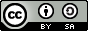# What is the mathematical significance of the constant C in an indefinite integral?

As we had seen in an earlier post, calculus bottleneck, calculus presents one of the most difficult topics for the students in higher mathematics. But the problem is not just limited to the students. Teachers feel it too. Too often the emphasis is given on how to solve integration and differentiation problems using “rules” and “methods” while the essence of what is happening is lost. Recently, I asked this question in an interview to a mathematics teacher who was teaching indefinite integration. This teacher had almost a decade of experience in teaching mathematics at +2 level. The teacher tried to answer this question by using an example of the function $$x^{2} + 5$$. Now when we take the derivative of this function, we get

$\dv{ (x^{2} + 5)}{x} = \dv{x^{2}}{x} + \dv{5}{x} = 2x$

as derivative of a constant (5 on our case) is 0). Now the teacher tried to argue, that integration is the reverse of the derivative), so

$\int 2x \, \dd x = \frac{2x^{2}}{2} + C = 2x + C$

After this the teacher tried to argue this $$C$$ represents the constant term (5) in our function $$x^{2} + 5$$. He tried to generalise the result, but he was thinking concretely in terms of the constant in the form of the numbers in the function. The teacher could understand the mechanism of solving the problem, but was not able to explain in clear mathematical terms, why the constant $$C$$ was required in the output of the indefinite integral. This difficulty, I think, partly arose because the teacher only thought in terms solving integrals and derivatives in a particular way, and knew about the connection between the two, but not in a deep way. He did in a way understood the essence of the constant $$C$$, but was not able to understand my question as a general question and hence replied only in terms of concrete functions. Even after repeated probing, the teacher could not get the essence of the question:

why do we add a constant term to the result of the indefinite integral?

To put it in another words, he was not able to generalise an abstract level of understanding from the examples that were discussed. The teacher was thinking only in terms of symbol manipulation rules which are sufficient for problem solving of these types. For example, look at the corresponding rules for differentiation and integration of the function $$x^{n}$$.

$\dv{x^{n}}{x} = n x^{n-1} \iff \int x^{n} \dd x = \frac{x^{n+1}}{n} + C$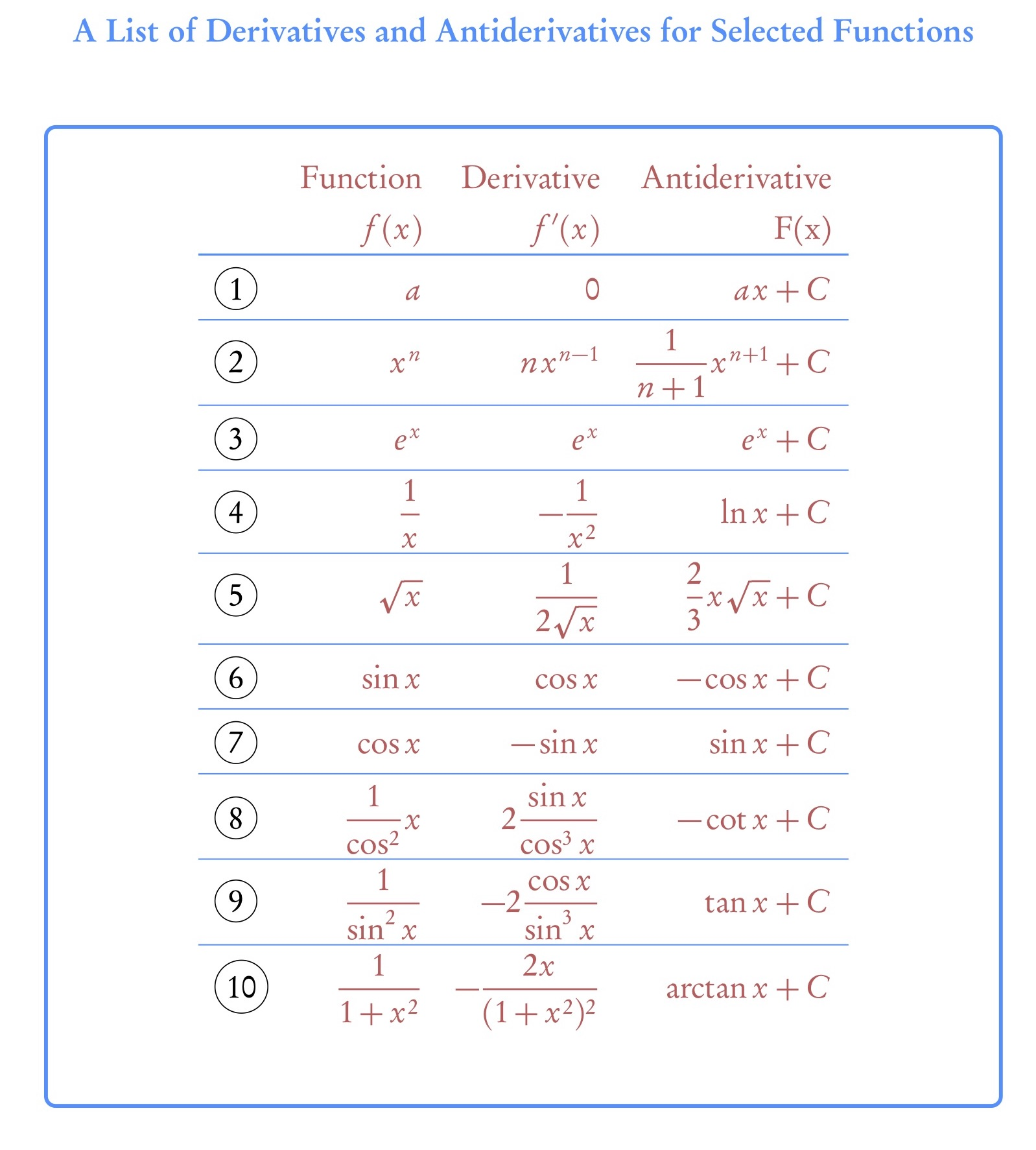Thus, we see according to above correspondence that adding any extra constant $$C$$ to the derivative formula will not affect it. So the teacher claimed it is this constant which appears in the integration rule as well. In a way this is a sort of correct explanation, but it does not get to the mathematical gist of why it is so. What is the core mathematical idea that this constant $$C$$ represents.

Another issue, I think, was the lack of any geometrical interpretation during the discussion. If you ask, what is the geometrical interpretation of the derivative you will get a generic answer along the lines: “It is the tangent to the curve” and for integration the generic answer is along the lines “It is the area under the curve”. Both these answers are correct, but how do these connect to the equivalence above? What is the relationship between the tangent to the curve and area under the curve which allows us to call the integral as the anti-derivative (or is the derivative an anti-integral?). I think to understand these concepts better we have to use the geometrical interpretation of the derivative and the integral from their first definitions.

The basic idea behind the derivative is that it represents the rate of change of a function $$f$$ at a given point. This idea, for an arbitrary function, can be defined (or interpreted) geometrically as:

The derivative of a function $$f$$ at a point $$x_{0}$$ is defined by the slope of the tangent to the graph of the function $$f$$ at the point  $$x = x_{0}$$.

The animation below shows how the slope of the tangent to the sine curve changes. Point $$B$$ in the animation below plots the $$(x, m)$$, where $$m$$ is the slope of the tangent for the given value of $$x$$. Can you mentally trace the locus of point $$B$$? What curve is it tracing?Now, the tangent to any point on a curve is unique. (Why is it so?) That means if one evaluates a derivative of a function at a point, it will be a unique result for that point.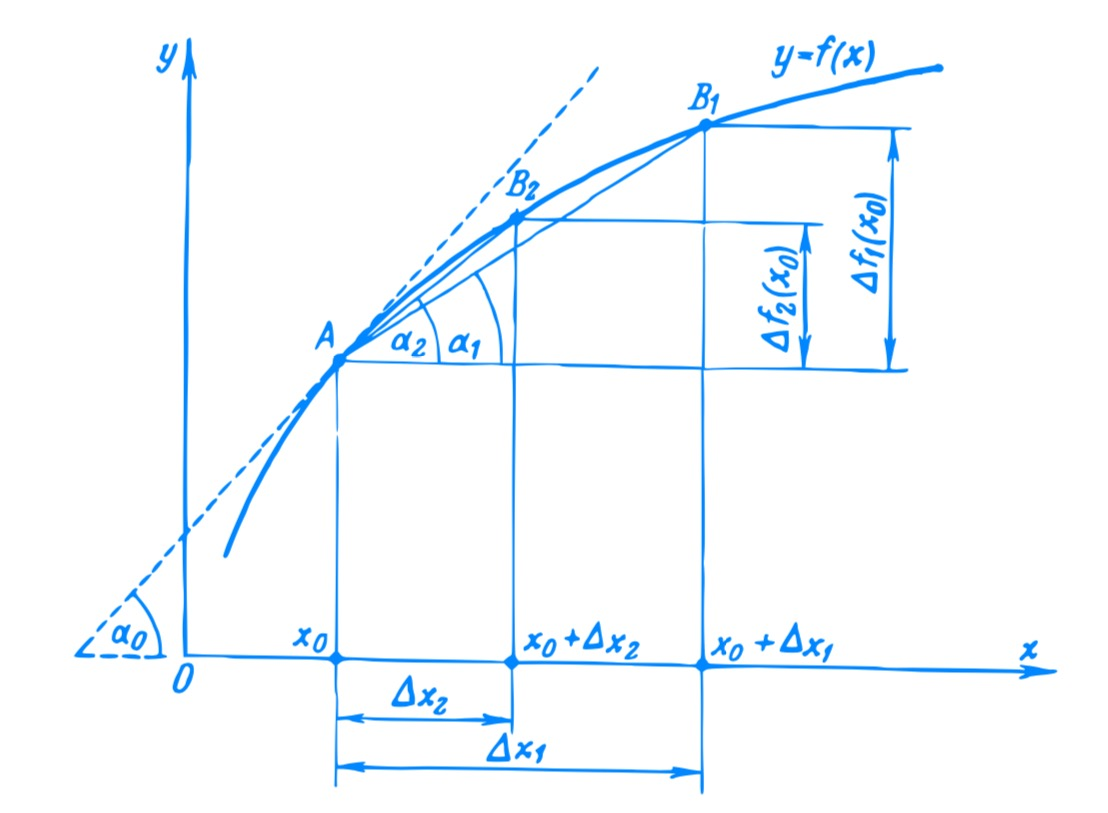This being cleared, now let us turn to the indefinite integral. One approach to understanding integration is to consider it as an inverse operation to the derivative, i.e. an anti-derivative.

An anti-derivative is defined as a function $$F(x)$$ whose derivative equals an initial function $$f (x)$$:

$f(x)= \dv{ F(x)}{x}$

Let us take an example of the function $$f(x) = 2x^{2} – 3x$$. The differentiation of this function gives us its derivative $$f'(x) = 4x – 3$$, and its integration gives us anti-derivative.
$F(x) = \frac{2}{3} x^{3} – \frac{3}{2} x^2$

This anti-derivative can be obtained by applying the known rules of differentiation in the reverse order. We can verify that the differentiation of the anti-derivative leads us to the original function.

$F'(x) = \frac{2}{3} 3 x^{2} – \frac{3}{2} 2 x = 2x^{2} – 3x$

Now if add a constant to the function $$F(x)$$, lets say number 4, we get a function $$G(x) = \frac{2}{3} x^{3} – \frac{3}{2} x^2 + 4$$. If we take the derivative of this function $$G(x)$$, we still get our original function back. This is due to the fact that the derivative of a constant is zero. Thus, there can be any arbitrary constant added to the function $$F(x)$$ and it will still be the anti-derivative of the original function $$f(x)$$.

An anti-derivative found for a given function is not unique. If $$F (x)$$ is an anti-derivative (for a function $$f$$ ), then any function $$F(x)+C$$, where $$C$$ is an arbitrary constant, is also an anti-derivative for the initial function because
$\dv{[F(x)+C]}{x} = \dv{ F(x)}{x} + \dv{ C}{x}= \dv{ F(x) }$

But what is the meaning of this constant $$C$$? This means, that each given function $$f (x)$$ corresponds to a family of anti-derivatives, $$F (x) + C$$. The result of adding a constant $$C$$ to any function is that it shifts along the $$Y$$-axis.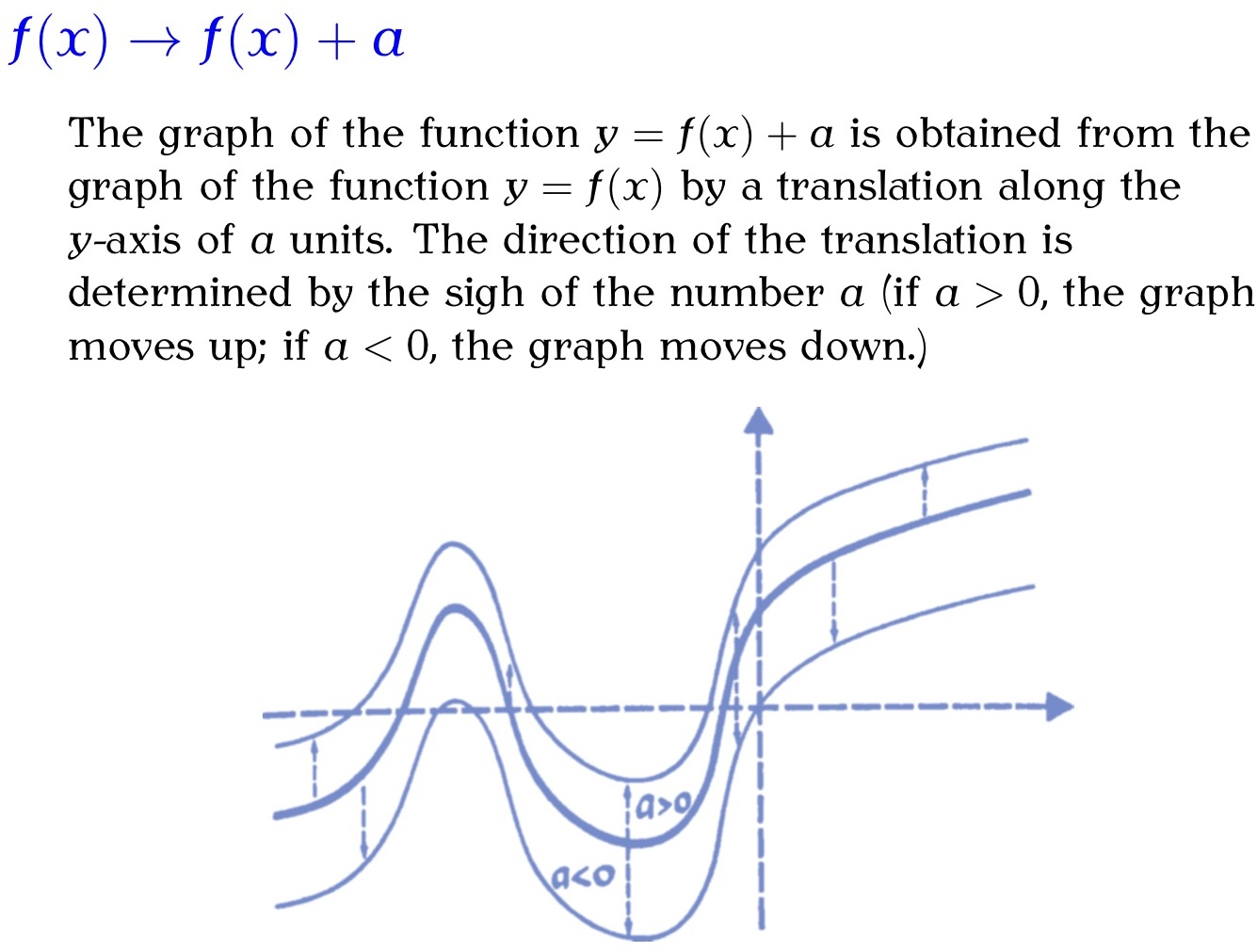Thus what it means for our case of result of the anti-derivative, the resultant would be a family of functions which are separated by $$C$$. For example, let us look at the anti-derivative of $$f (x) = \sin x$$. The curves of anti-derivatives for this function are plotted in will be of the form

$F ( x ) = − \cos x + C$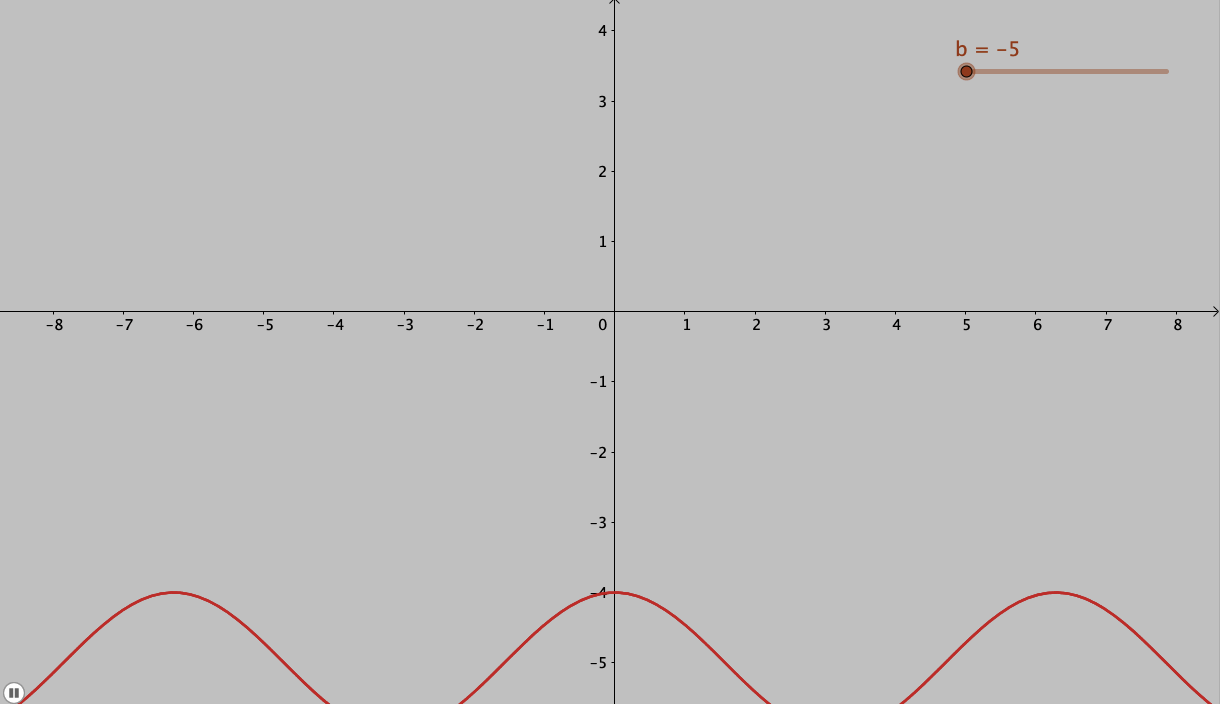A family of curves of the anti-derivatives of the function $$f (x) = \sin x = – \cos x$$

And this is the reason for adding the arbitrary constant $$C$$ to our result of the anti-derivative: we get a family of curves and the solution is not unique.

Now can we ever know the value of $$C$$? Of course we can, but for this we need to know the some other information about the problem at hand. These can be initial conditions (values) of the variables or the boundary condition. Once we know these we can determine a particular curve (particular solution) from the family of curves for that given problem.

Lev Tarasov – Calculus – Basic Concepts For High Schools (Starts with and explains  the basic mathematical concepts required to understand calculus. The book is in the form of a dialogue between the author and the student, where doubts, misconceptions and aha moments are discussed.)

Morris Kline – Calculus – A physical and intuitive approach (Builds the concepts in the context of the physical problems that calculus was invented to solve.  A book every physics student should read to get an understanding of how mathematics helps solve physical problems.)

Richard  Courant and Fritz John – Introduction to Calculus Analysis (In 2 Volumes) (Standard college level text with in-depth discussions. First volume is rigorous with basic concepts required to conceptually understand the topics and their applications/implications.)

# An example use of H5P item for creating hotspots

Just trying out different H5P items. This item has a flower  and hotspots indicating parts of the flower.

Click on the question marks to know more..

# Interesting LaTeX Packages – Drawing the Solar System

Many times we need to quickly illustrate the solar system or the planets. Usually we use photos to illustrate the planets. But sometimes the photos can be an over kill. Also to draw the entire solar system is a typical and can be used for illustrative purposes. Though most of the illustrations of the solar system in the school and other text books are horribly out of scale, (with no indication that the figure is not to scale!). For example,  look at the illustration in the Class 6 Science Textbook from NCERT.A good visualisation will always present a scale, and/or indicate whether the visualisation is to the scale or not. Though in the visualisation above the distances are given, they are not to scale.

Coming back to the topic of our post, a simple way to draw solar system diagram in latex is to use the PStricks package solarsystem. The package can create “Position of the visible planets, projected on the plane of the ecliptic” at a given time and date. This feature might be useful sometimes.

From the package manual:

As we can not represent all the planets in the real proportions, only Mercury, Venus, Earth and Mars are the proportions of the orbits and their relative sizes observed. Saturn and Jupiter are in the right direction, but obviously not at the right distance.
The orbits are shown in solid lines for the portion above the ecliptic and dashed for the portion located below.

The use of the command is very simple, just specify the date of observation with the following parameters, for example:
\SolarSystem[Day=31,Month=12,Year=2020,Hour=23,Minute=59,Second=59]
By default, if no parameter is specified, \SolarSystem gives the configuration day 0 hours to compile.

The resulting output for the above code: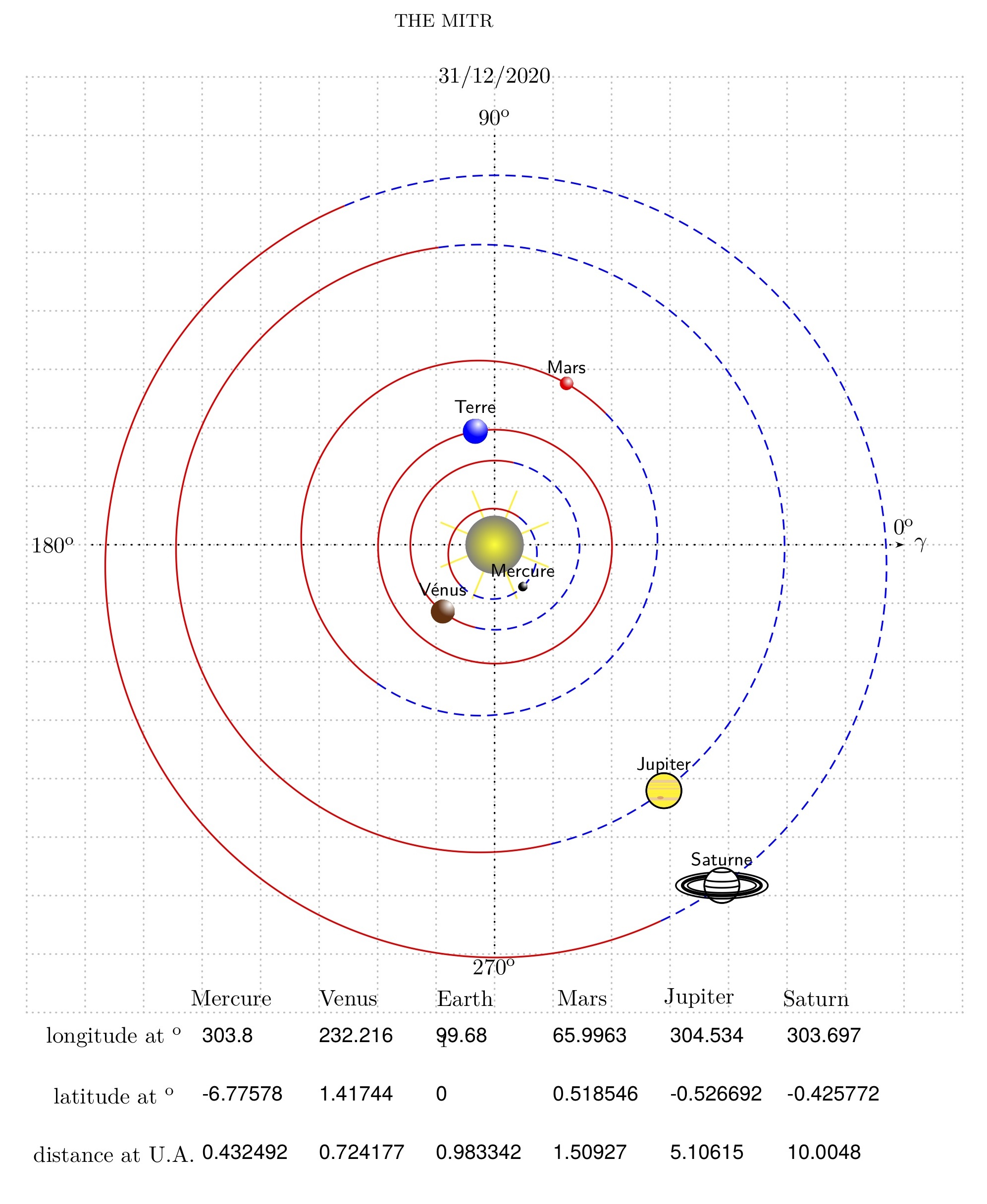The output also provides the longitude and latitude of the planets at the time given.

Another package that is useful to create free standing planets is the tikz-planets package which we will see next.

# John Tukey on data based pictures and graphs

John Tukey‘s wisdom on importance and value of graphics and pictures in making sense of exploring data.

Consistent with this view, we believe, is a clear demand that pictures based on exploration of data should force their messages upon us. Pictures that emphasize what we already know — “security blankets” to reassure us — are frequently not worth the space they take. Pictures that have to be gone over with a reading glass to see the main point are wasteful of time and inadequate of effect. The greatest value of a picture is when it forces us to notice what we never expected to see. (p. vi emphasis in original)

John Tukey – Exploratory Data Analysis

# Galileo’s Experiments on Accelerated Motion

A short account of Galileo’s description of his own experiment on accelerated motion — a short account of it, the apparatus he used and the results he got.
The first argument that Salviati proves is that in accelerated motion the change in velocity is in proportion to the time (𝑣 ∝ 𝑡) since the motion began, and not in proportion to the distance covered (𝑣 ∝ 𝑠) as is believed by Sargedo.

“But for one and the same body to fall eight feet and four feet in the same time is possible only in the case of instantaneous (discontinuous) motion; but observation shows us that the motion of a falling body occupies time, and less of it in covering a distance of four feet than of eight feet; therefore it is not true that its velocity increases in proportion to the space. (Salviati)

Also, he proves that the increase in proportion is not of simple doubling but larger. They agree upon a definition of uniformly accelerated motion,

“A motion is said to be equally or uniformly accelerated when, starting from rest, its momentum receives equal increments in equal times. (Sargedo)

To this definition Salviati adds an assumption about inclined planes, this assumption is that for a given body, the increase in speed while moving down the planes of difference inclinations is equal to the height of the plane. This also includes the case if the body is dropped vertically down, it will still gain the same speed at end of the fall as it would gain from rolling on the incline This assumption makes the final speed independent on the profile of the incline. For example, in the figure below, the body falling along𝐶 → 𝐵, 𝐶 → 𝐷 and 𝐶 → 𝐴 will attain the same final speed.This result is also proved via a thought experiment (though it might be feasible to do this experiment) for a pendulum. The pendulum rises to the height it was released from and not more.
After stating this theorem, Galileo then suggests the experimental verification of the theorem. of The actual apparatus that Galileo uses is an wooden inclined slope of following dimensions: length 12 cubits (≈ 5.5 m, 1 cubit ≈ 45.7 cm), width half-cubit and three-finger breadths thick . In this plank of wood, he creates a very smooth groove which is about a finger thick. (What was the thickness of Galileo’s fingers?) The incline of this plank are changed by lifting one end. A bronze ball is rolled in this groove and time taken for descent is noted.

“We repeated this experiment more than once in order to measure the time with an accuracy such that the deviation between two observations never exceeded one- tenth of a pulse-beat.

Then Galileo performed variations in the experiment by letting the ball go different lengths (not full) of the incline and “found that the spaces traversed were to each other as the squares of the times, and this was true for all inclinations of the plane”. Each variation was repeated hundreds of times so as to rule out any errors. Also, the fact that for different inclines the times of descent were in noted and were in agreement with the predictions.
Since there were no second resolution clocks to measure time, Galileo devised a method to measure time using water. This was not new, water clocks were used earlier also.

The basic idea was to the measure the amount of water that was collected from the start of the motion to its end. The water thus collected was weighed on a good balance.This weight of water was used as a measure of the time. A sort of calibration without actually measuring the quantity itself: “the differences and ratios of these weights gave us the differences and ratios of the times”

Galileo used a long incline, so that he could measure the time of descent with device he had. If a shorted incline was used, it would have been difficult to measure the shorter interval of time with the resolution he had. Measuring the free fall directly was next to impossible with the technology he had. Thus the extrapolation to the free fall was made continuing the pattern that was observed for the “diluted” gravity.

“You present these recondite matters with too much evidence and ease; this great facility makes them less appreciated than they would be had they been presented in a more abstruse manner. For, in my opinion, people esteem more lightly that knowledge which they acquire with so little labor than that acquired through long and obscure discussion. (Sargedo)

### Reference

Dialogues Concerning Two New Sciences

# Bertrand Russel’s proof of naïve realism being false

What is naïve realism you may ask? To put simply naïve realism is a belief that whatever you see with your senses is the reality. There is nothing more to reality than what your sense perceptions bring to you. It is a direct unmediated access to reality. There is no “interpretation” involved.

In philosophy of perception and philosophy of mind, naïve realism (also known as direct realism, perceptual realism, or common sense realism) is the idea that the senses provide us with direct awareness of objects as they really are. When referred to as direct realism, naïve realism is often contrasted with indirect realism.

Naïve Realism

To put this in other words, naïve realism fails to distinguish between the phenomenal and the physical object. That is to say, all there is to the world is how we perceive it, nothing more.

Bertrand Russel gave a one line proof of why naïve realism is false. And this is the topic of this post. Also, the proof has some implications for science education, hence the interest.

Naive realism leads to physics, and physics, if true, shows that naive realism is false. Therefore naive realism, if true, is false; therefore it is false.

As quoted in Mary Henle – On the Distinction Between the Phenomenal and the Physical Object, John M. Nicholas (ed.), Images, Perception, and Knowledge, 187-193. (1977)

Henle in her rather short essay (quoted above) on this makes various philosophically oriented arguments to show that it is an easier position to defend when we make a distinction between the two.

But considering the “proof” of Russel, I would like to bring in evidence from science education which makes it even more compelling. There is a very rich body of literature on the theme of misconceptions or alternative conceptions among students and even teachers. Many of these arise simply because of a direct interpretation of events and objects around us.

Consider a simple example of Newton’s first law of motion.

In an inertial frame of reference, an object either remains at rest or continues to move at a constant velocity, unless acted upon by a force.

Now for the naïve realists this will never be possible, as they will never see an object going by itself without application of any force. In real world, friction will bring to halt bodies which are moving. Similar other examples from the misconceptions also do fit in this pattern. This is perhaps so because most of the science is counter-intuitive in nature. With our simple perception we can only do a limited science (perhaps create empirical laws). So one can perhaps say that learners with alternative conceptions hold naïve realist world-view (to some degree) and the role of science education is to change this.

# Experiments, Data and Analysis

There are many sad stories of students, burning to carry out an experimental project, who end up with a completely unanalysable mishmash of data. They wanted to get on with it and thought that they could leave thoughts of analysis until after the experiment. They were wrong. Statistical analysis and experimental design must be considered together…
Using statistics is no insurance against producing rubbish. Badly used, misapplied statistics simply allow one to produce quantitative rubbish rather than qualitative rubbish.
–  Colin Robson (Experiment, Design and Statistics in Psychology)